# Horocycle flow

(diff) ← Older revision | Latest revision (diff) | Newer revision → (diff)

A flow in the space of bihedra of an-dimensional Riemannian manifold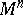(usually closed) for which the concept of a horocycle is defined; the horocycle flow describes the movement of the bihedra along the horocycles which they define.

The basic cases in which the concept of a horocycle is defined are those in which the curvature of the Riemannian metric is negative, and eitheror the curve is constant. With a bihedron, i.e. an orthonormal-frame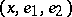(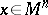;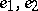are mutually orthogonal unit tangent vectors at the point) is associated the horocycle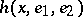throughin the direction of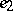. It is situated on the horosphere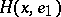through, the-dimensional manifold orthogonal to the family of geodesic lines, asymptotic (in the positive direction) to the geodesic line which passes throughin the direction of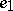. The direction ondefined byis taken to be positive (in casethis is the only role of;andcan have self-intersections; the simplest way to avoid the ambiguity which can arise from this is to carry out analogous constructions not in, but in its universal covering manifold — when the curvature is constant, this is the ordinary-dimensional Lobachevskii space — and to project the horocycle obtained there into). Under the action of a horocycle flow, the bihedronduring timepasses to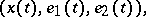where, whenincreases, moves at unit velocity alongin the positive direction, the unit vector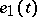is orthogonal toat the point(the choice of one of the two possible directions foris made by continuity) and.

Horocycle flows were studied because they played an important role in the research of geodesic flows (cf. Geodesic flow) on manifolds of negative curvature . Now this role is played by certain foliations (cf. Foliation) arising from the theory of-systems (cf.-system), and horocycle flows became a research subject in its own right. The properties of a horocycle flow have been well established (see , ). For various generalizations, see –.

How to Cite This Entry:
Horocycle flow. Encyclopedia of Mathematics. URL: http://encyclopediaofmath.org/index.php?title=Horocycle_flow&oldid=15745
This article was adapted from an original article by D.V. Anosov (originator), which appeared in Encyclopedia of Mathematics - ISBN 1402006098. See original article